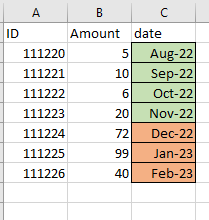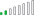cancel
Showing results for
Did you mean:Helper II

## calculate value except last 3 months

Hi All,

I want to calculate value except last 3 months date.

example:  as per below screenshot i need to exclude last 3 months date.(means latest 3 months should be excluded)output:Thanks,

Varan

1 ACCEPTED SOLUTIONResolver II

Try this,

``````Count Except Last 3 Months =
SUMX(
FILTER(
Sample_Data_Table,
),
Sample_Data_Table[Amount]
)``````

This will give you following resultResolver II

Try this,

``````Count Except Last 3 Months =
SUMX(
FILTER(
Sample_Data_Table,
),
Sample_Data_Table[Amount]
)``````

This will give you following result